# MATHEMATICS —2004 (Set II— Delhi)

Except for the following questions, all the remaining questions have been asked in Set I.

Q. 3. Solve for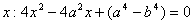Ans.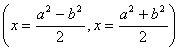Q. 4. The 7 th term of an A.P. is 20 and its 13 th term is 32. Find the A.P.

Ans. (8, 10, 12, 14, ...)

Q. 7. A loan of Rs.21,600 has to be repaid in two equal annual instalments. If the interest is charged at the rate of 16% per annum, compounded annually, find the amount of each instalment.

Ans. (Rs.13,456)

Q. 8. A radio is available for Rs.1,500 cash or Rs.300 as cash down payment followed by three equal monthly instalments of Rs.420. Find the rate of interest charged under the instalment scheme.

Ans.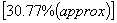Q. 11. Solve for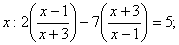given thatAns.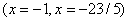Q. 14. A solid metallic sphere of diameter 28 cm is melted and recasted into a number of smaller cones, each of diametercm and height 3 cm. Find the number of cones so formed.

Ans.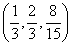Q. 20. A bag contains 7 black, 5 red and 3 white balls. A ball is drawn from the bag at random. Find the probability that the ball drawn is (i) red (ii) black or white (iii) not black.

Ans.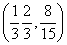Q. 24. Manmohan has an annual income of Rs.3,40,000 (exclusive of HRA). He contributes Rs.5,000 per month towards his GPF and pays an annual LIC premium of Rs.15,000. He has invested Rs.20,000 in N.S.C's. He pays Rs. 4,200 as income tax per month for the first 11 months. Find his income tax liability for the last month of the financial year.

Use the following for calculating income tax:

 (a) Standard Deduction Gross income Deduction (i) Upto Rs.75,000 (ii) From Rs.75,001 to Rs.5,00,000 (iii) More than Rs,5,00,000 40% of gross income Rs.30,000 Rs.20,000 (b) Rates of Taxes Slab Income tax (i) Upto Rs. 50,000 (ii) From Rs. 50,001 to Rs. 60,000 (iii) From Rs. 60,001 to Rs. 1,50,000 (iv) Rs. 1.50,000 and above No tax 10% of the amount exceeding Rs.50,000 Rs.1,000+20% of the amount Exceeding Rs.60,000 Rs.19,000+30% of the amount Exceeding Rs.1,50,000 (c) Rebate in Tax (i) 20% of the savings subject to a maximum of Rs. 14,000 if the gross income is upto Rs. 1,50,000. (ii)15% of the savings subject to a maximum of Rs. 10,500 if the gross income is from Rs. 1,50,001 to Rs. 5,00,000.

Ans.(Rs.10,300)

Maths 2004 Question Papers Class X
CBSE 2004 Question Papers Class X